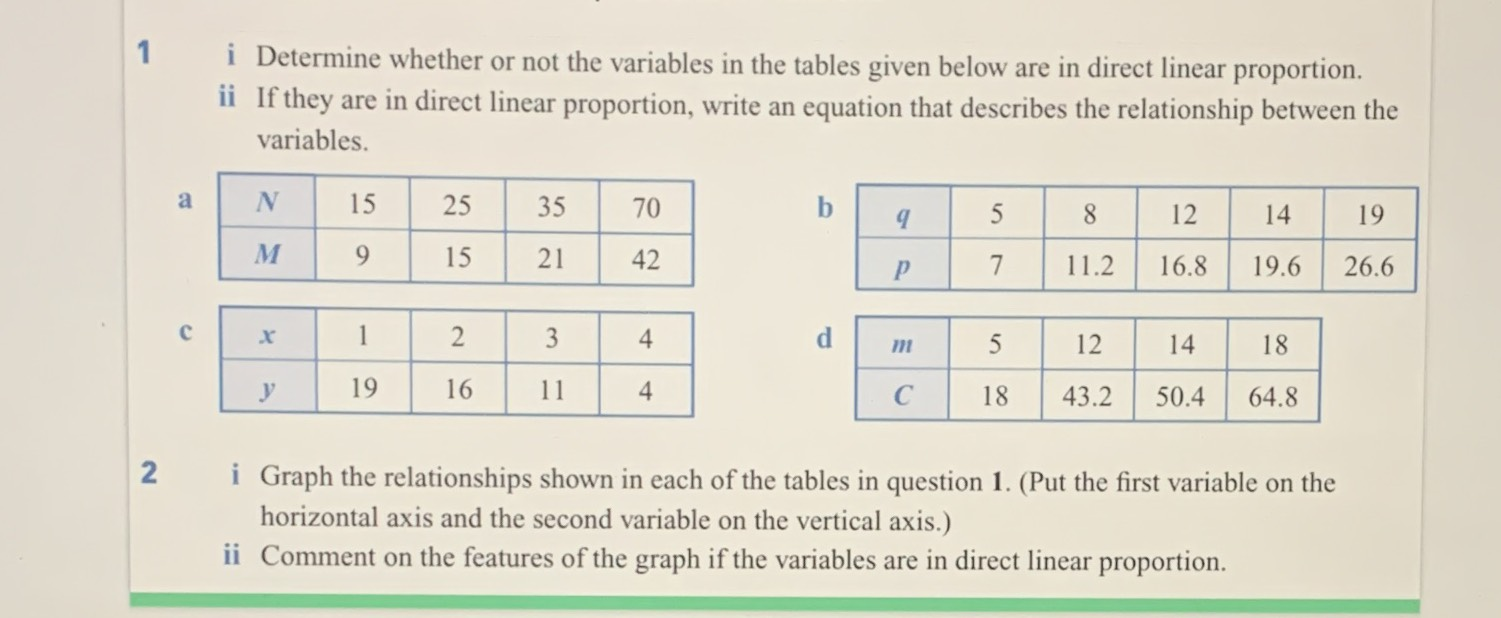### ¿Todavía tienes preguntas de matemáticas?

Pregunte a nuestros tutores expertos
Algebra
Pregunta$$1$$ i Determine whether or not the variables in the tables given below are in direct linear proportion.

ii If they are in direct linear proportion, write an equation that describes the relationship between the variables.

a. $$M = 0.6N$$

b. $$p = 1.4q$$

c. $$non- linear$$

d. $$C = 3.6m$$

Solución
View full explanation on CameraMath App.